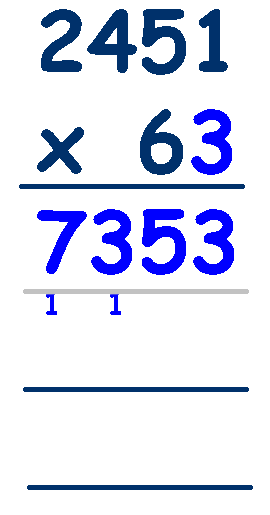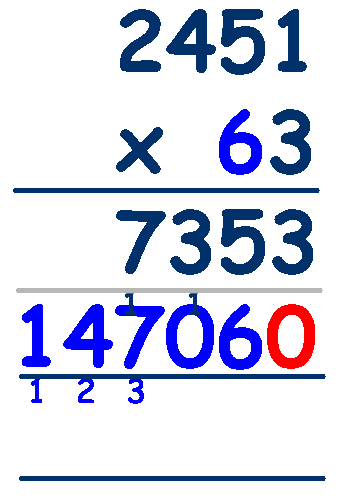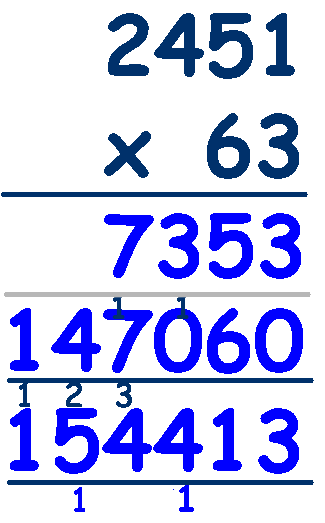# Decimals - Multiplying Decimal Numbers

### To multiply decimal numbers we can use the methods shown in Written Methods of Multiplication , but we must remember to account for the position of the decimal points.

The first step is to convert any decimal numbers into whole numbers. We do this by multiplying the decimal number by amounts of 10. To convert a decimal number with one decimal place, say 2.4, we need to multiply by 10 (by moving the entire number one place to the left - see 'Place Value'). For a number with 2 decimal places, say 5.26, we need to multiply by 100 (by moving the entire number two places to the left - see 'Place Value').

## Lets take an example:   4.2 x 3

(Estimate first - 4 x 3= 12 ... so we know the result should be around 12)

Step 1 - convert 4.2 to a whole number by multiplying by 10 ... 4.2 x 10 = 42

Step 2 - do the calculation 42 x 3 (using either method - grid or standard) = 126

Step 3 - reverse the effect of multiplying and return our answer to whole numbers and decimal fractions by dividing by the same amount that we used to convert to whole numbers - in other words do the inverse. 126 ÷ 10 = 12.6 (by moving the entire number one place to the right - see 'Place Value').

4.2 x 3 = 12.6 ... Check with our estimate of around 12 - close enough - OK

## Lets look at a harder example:   2.4 x 3.6

(Estimate first - 2 x 4= 8 - so we know the result should be around 8)

Step 1 - convert 2.4 to a whole number by multiplying by 10 ... 2.4 x 10 = 24
and convert 3.6 to a whole number by multiplying by 10 ... 3.6 x 10 = 36

Step 2 - do the calculation 24 x 36 (using either method - grid or standard) = 864

Step 3 - reverse the effect of multiplying and return our answer to whole numbers and decimal fractions by dividing by the same amount that we used to convert to whole numbers. In this case we multiplied by 10 twice, which is the same as multiplying by 100, so we need to divide by 100 ... 864 ÷ 100 = 8.64 (move 2 places to the right).

2.4 x 3.6 = 8.64 - Check with our estimate of around 8 - close enough - OK

Once your child has a good understanding of what is happening by converting to whole numbers, making the calculation and then converting back you can speed up the process by first performing the calculation on the numbers without worrying about the decimal points. Then count how many digits are to the right of the decimal point in both numbers and insert a decimal point that many digits, starting at the right of the answer.

### Sounds confusing so lets try an example, say, 24.51 x 6.3

(Estimate first - 25 x 6= 150 - so we know the result should be around 150)

Multiply by the units digit, 3Multiply the tens digit, 6

Don't forget to include the 0,
because it is actually 60Check with our estimate of around 150 - nowhere near - What's wrong?

### We need to remember the decimal points or our answer will be way out!

Look at our original numbers, 24.51 has 2 digits to the right of the decimal point and 6.3 has 1 digit to the right of the decimal point. So that's three places.

We need to insert the decimal point in the answer, at 3 places starting from the right.

## So 154413 becomes 154.413

Check with our estimate of around 150 - close enough - OK

The reason this works is because what we are really doing is multiplying the original numbers by varying orders of ten to make them whole numbers. After performing the multiplication we need to do the inverse (divide by the same amount) to reverse the effect of multiplying and return our answer to whole numbers and decimal fractions.
This is why making an estimation at the beginning is important.

Try these worksheets from Maths is Fun